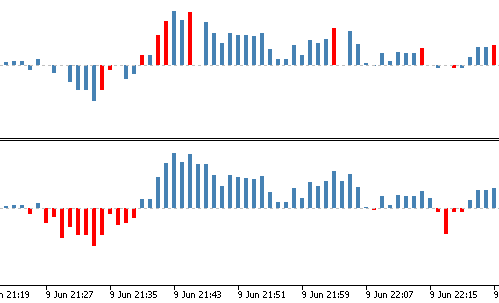Indicator color issue747

Hi, I'm experiencing a strange thing with a colorized indicator histogram. The histogram is defined like so:

```#property indicator_type1   DRAW_COLOR_HISTOGRAM
#property indicator_color1  clrSteelBlue,clrRed
#property indicator_style1  STYLE_SOLID
#property indicator_width1  2```

Note the two colors.

In the chart I get a blue histogram, which is fine, but occasionally it shows red bars. And also some bars are not showing although their value is different from zero, like the one highlighted with the mouse in the picture.

Could someone explain this to me?38403

Impossible to answer without seeing the related code.747

Alain Verleyen:
Impossible to answer without seeing the related code.

Here's the full indicator. The original had Black instead of Red as 2nd color, I changed that to highlight the bars in question.

```//+------------------------------------------------------------------+
//|                                             Balance of Power.mq5 |
//|                                            http://www.robofx.org |
//+------------------------------------------------------------------+
#property version   "1.00"

#property indicator_separate_window
#property indicator_buffers 2
#property indicator_plots   1
//--- plot BoP
#property indicator_label1  "BOP"
#property indicator_type1   DRAW_COLOR_HISTOGRAM
#property indicator_color1  clrSteelBlue,clrRed
#property indicator_style1  STYLE_SOLID
#property indicator_width1  2

#include <MovingAverages.mqh>    //--- include moving averages methods

input int BOP_Period = 14;       //--- smoothing period

//--- indicator buffers
double         Buffer1[];        //--- raw data buffer
double         BOP[];            //--- main indicator buffer
//+------------------------------------------------------------------+
//| Custom indicator initialization function                         |
//+------------------------------------------------------------------+
int OnInit()
{
//--- setting dynamic arrays as indicator buffers
SetIndexBuffer(0,BOP,INDICATOR_DATA);
SetIndexBuffer(1,Buffer1,INDICATOR_CALCULATIONS);

//--- setting the accuracy for indicator values
IndicatorSetInteger(INDICATOR_DIGITS,_Digits);

// --- setting the indicator name to be displayed in a separate subwindow
IndicatorSetString(INDICATOR_SHORTNAME,"Balance of Power ("+string(BOP_Period)+")");
//---
return(0);
}
//+------------------------------------------------------------------+
//| Custom indicator iteration function                              |
//+------------------------------------------------------------------+
int OnCalculate(const int rates_total,
const int prev_calculated,
const datetime &time[],
const double &open[],
const double &high[],
const double &low[],
const double &close[],
const long &tick_volume[],
const long &volume[],
{
//---
int start;

//--- check for data
if(rates_total < BOP_Period) return(0);

//--- calculations of the starting number limit for the bar recalculation loop
//--- recalculate the last bar
if(prev_calculated > 1) start = prev_calculated-1;
else
{
start = 1;
}

//--- main indicator calculation loop
for(int i=start;i<rates_total && !IsStopped();i++)
{
//--- avoid zero divide problem in case high == low
if((high[i]-low[i]) > 0.0)
{
//--- calculate raw balance of power for the i-th bar
Buffer1[i] = (close[i]-open[i])/(high[i]-low[i]);
}
else Buffer1[i] = 0;
}

// --- smoothing the indicator data using simple moving average
SimpleMAOnBuffer(rates_total,prev_calculated,0,BOP_Period,Buffer1,BOP);

//--- return value of prev_calculated for next call
return(rates_total);
}```38403

lippmaje:

Here's the full indicator. The original had Black instead of Red as 2nd color, I changed that to highlight the bars in question.

You are using a color histogram which needs 2 buffers, but you didn't declare or set a colors buffer. The colors are aleatory.Files:747